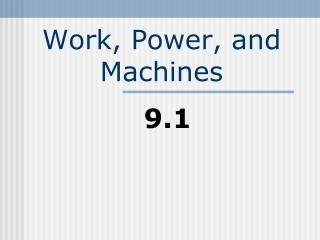# Work, Power, and Machines - PowerPoint PPT PresentationDownload PresentationWork, Power, and Machines

Work, Power, and MachinesDownload Presentation## Work, Power, and Machines

- - - - - - - - - - - - - - - - - - - - - - - - - - - E N D - - - - - - - - - - - - - - - - - - - - - - - - - - -
##### Presentation Transcript

1. Work • A quantity that measures the effects of a force acting over a distance • Work = force x distance • W = Fd

2. Work • Work is measured in: • Nm • Joules (J)

3. Work Example • A crane uses an average force of 5200 N to lift a girder 25 m. How much work does the crane do?

4. Work Example • Work = Fd • Work = (5200 N)(25m) • Work = 130000 N  m = 130000 J

5. Power • A quantity that measures the rate at which work is done • Power = work/time • P = W/t

6. Power • Watts (W) is the SI unit for power • 1 W = 1 J/s

7. Power Example • While rowing in a race, John uses 19.8 N to travel 200.0 meters in 60.0 s. What is his power output in Watts?

8. Power Example • Work = Fd • Work = 19.8 N x 200.0 m= 3960 J • Power = W/t • Power = 3960 J/60.0 s • Power = 66.0 W

9. Machines • Help us do work by redistributing the force that we put into them • They do not change the amount of work

10. Machines • Change the direction of an input force (ex car jack)

11. Machines • Increase an output force by changing the distance over which the force is applied (ex ramp) • Multiplying forces

12. Mechanical Advantage • A quantity that measures how much a machine multiples force or distance.

13. Mechanical Advantage Inputdistance Mech. Adv = Output Distance Output Force Mech. Adv. = Input Force

14. Mech. Adv. example • Calculate the mechanical advantage of a ramp that is 6.0 m long and 1.5 m high.

15. Mech. Adv. Example • Input = 6.0 m • Output = 1.5 m • Mech. Adv.=6.0m/1.5m • Mech. Adv. = 4.0

16. Simple Machines • Most basic machines • Made up of two families • Levers • Inclined planes

17. The Lever Family • All levers have a rigid arm that turns around a point called the fulcrum.

18. The Lever Family • Levers are divided into three classes • Classes depend on the location of the fulcrum and the input/output forces.

19. First Class Levers • Have fulcrum in middle of arm. • The input/output forces act on opposite ends • Ex. Hammer, Pliers

20. First Class Levers Input Force Output Force Fulcrum

21. Second Class Levers • Fulcrum is at one end. • Input force is applied to the other end. • Ex. Wheel barrow, hinged doors, nutcracker

22. Second Class Levers Output Force Fulcrum Input Force

23. Third Class Levers • Multiply distance rather than force. • Ex. Human forearm

24. Third Class Levers • The muscle contracts a short distance to move the hand a large distance

25. Third Class Levers Output distance Input Force Fulcrum

26. Pulleys • Act like a modified member of the first-class lever family • Used to lift objects

27. Pulleys Output Force Input force

28. The Inclined Plane • Incline planes multiply and redirect force by changing the distance • Ex loading ramp

29. The Inclined Plane • Turns a small input force into a large output force by spreading the work out over a large distance

30. A Wedge • Functions like two inclined planes back to back

31. A Wedge • Turns a single downward force into two forces directed out to the sides • Ex. An axe , nail

32. Or Wedge Antilles from Star Wars

33. Not to be mistaken with a wedgIEEEEE

34. A Screw • Inclined plane wrapped around a cylinder

35. A Screw • Tightening a screw requires less input force over a greater distance • Ex. Jar lids

36. Compound Machines • A machine that combines two or more simple machines • Ex. Scissors, bike gears, car jacks